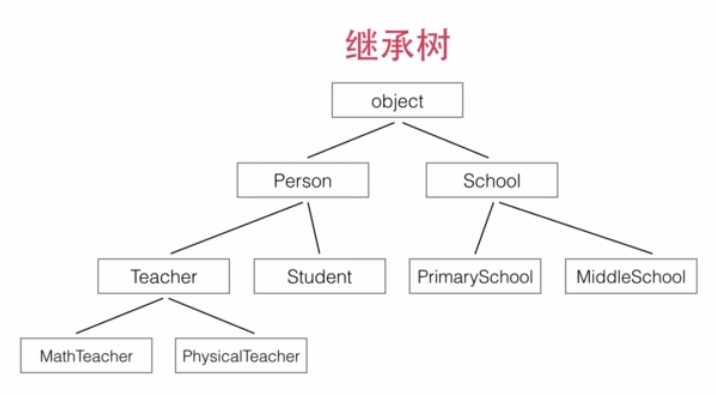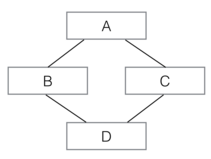# [python进阶课程] 面向对象编程

http://www.imooc.com/learn/317

# 模块和包

: 文件夹 (可以有多级), 且包含`__init__.py`文件(每层都要有) 模块: py文件

### 导入模块

```from math import log
from logging import log as logger
```

### 动态导入模块

```try:
from cStringIO import StringIO
except ImportError:
from StringIO import StringIO
```

### 使用__future__

Python的新版本会引入新的功能，但是，实际上这些功能在上一个老版本中就已经存在了。要“试用”某一新的特性，就可以通过导入__future__模块的某些功能来实现。

ex. 在Python 2.7中引入3.x的除法规则，导入__future__的division：

```>>> from __future__ import division
>>> print 10 / 3
3.3333333333333335
```

• easy_install
• pip (推荐)

# 面向对象编程基础

OOP: 数据的封装

### 初始化实例属性

`setattr``__init__`接受任意的kw参数:

`setattr(object, name, value)`
This is the counterpart of getattr(). The arguments are an object, a string and an arbitrary value. The string may name an existing attribute or a new attribute. The function assigns the value to the attribute, provided the object allows it. For example, setattr(x, 'foobar', 123) is equivalent to x.foobar = 123.

```class Person(object):
def __init__(self, name, gender, birth, **kw):
self.name = name
self.gender = gender
self.birth = birth
for k, v in kw.iteritems():
setattr(self, k, v)
```

### 访问限制

Python对属性权限的控制是通过属性名来实现的.

• 如果一个属性由双下划线开头(`__`)，该属性就无法被外部访问。
• 但是，如果一个属性以"`__xxx__`"的形式定义，那它又可以被外部访问了，以"`__xxx__`"定义的属性在Python的类中被称为特殊属性有很多预定义的特殊属性可以使用，通常我们不要把普通属性用"xxx"定义。
• 以单下划线开头的属性"`_xxx`"虽然也可以被外部访问，但是，按照习惯，他们不应该被外部访问。

### 创建类属性

```class Person(object):
def __init__(self, name):
self.name = name
```

```print Person.address
```

### 定义类方法

```class Person(object):
count = 0
@classmethod
def how_many(cls):
return cls.count
def __init__(self, name):
self.name = name
Person.count = Person.count + 1
print Person.how_many()
p1 = Person('Bob')
print Person.how_many()
```

# 类的继承python的继承:

• 总是从某个类继承(最上层是`object`)
• 不要忘记`super.__init__`调用

super(SubCls, self)将返回当前类继承的父类, 注意self参数已在super()中传入，在__init__()中将隐式传递，不需要写出（也不能写）。
def init(self, args):
super(SubCls, self).init(args)
pass

### 判断类型

```>>> isinstance(p, Person)
True    # p是Person类型
>>> isinstance(p, Student)
False   # p不是Student类型
>>> isinstance(p, Teacher)
False   # p不是Teacher类型
>>> isinstance(s, Person)
True    # s是Person类型
```

### 多态

```class Book(object):
def whoAmI(self):
return 'I am a book'
```

### 多重继承

```class A(object):
def __init__(self, a):
print 'init A...'
self.a = a

class B(A):
def __init__(self, a):
super(B, self).__init__(a)
print 'init B...'

class C(A):
def __init__(self, a):
super(C, self).__init__(a)
print 'init C...'

class D(B, C):
def __init__(self, a):
super(D, self).__init__(a)
print 'init D...'
```D 同时继承自 B 和 C，也就是 D 拥有了 A、B、C 的全部功能。多重继承通过 super()调用__init__()方法时，A 虽然被继承了两次，但`__init__()`只调用一次：

```>>> d = D('d')
init A...
init C...
init B...
init D...
```

### 获取对象信息

```>>> type(123)
<type 'int'>
>>> s = Student('Bob', 'Male', 88)
>>> type(s)
<class '__main__.Student'>
```

```>>> dir(123)   # 整数也有很多属性...
['__abs__', '__add__', '__and__', '__class__', '__cmp__', ...]

>>> dir(s)
['__class__', '__delattr__', '__dict__', '__doc__', '__format__', '__getattribute__', '__hash__', '__init__', '__module__', '__new__', '__reduce__', '__reduce_ex__', '__repr__', '__setattr__', '__sizeof__', '__str__', '__subclasshook__', '__weakref__', 'gender', 'name', 'score', 'whoAmI']
```

`dir()`返回的属性是字符串列表，如果已知一个属性名称，要获取或者设置对象的属性，就需要用 `getattr()``setattr()`函数了：

```>>> getattr(s, 'name')  # 获取name属性
'Bob'
>>> setattr(s, 'name', 'Adam')  # 设置新的name属性
>>> s.name
>>> getattr(s, 'age')  # 获取age属性，但是属性不存在，报错：
Traceback (most recent call last):
File "<stdin>", line 1, in <module>
AttributeError: 'Student' object has no attribute 'age'
>>> getattr(s, 'age', 20)  # 获取age属性，如果属性不存在，就返回默认值20：
20

class Person(object):
def __init__(self, name, gender, **kw):
for k,v in kw.iteritems():
setattr(self, k, v)

p = Person('Bob', 'Male', age=18, course='Python')
print p.age
print p.course
```

# 定制类

### 特殊方法

• 定义在class中
• 不需要直接调用: py的函数或操作符会自动调用

ex. 任何数据类型的实例都有`__str__()`特殊方法.

pothon的特殊方法:

• `__str__`: 用于print
• `__len__`: 用于len
• `__cmp__`: 用于比较`cmp`/排序`sorted`

### str 和 repr

```>>> p = Person('Bob', 'male')
>>> print p
(Person: Bob, male)
>>> p
<main.Person object at 0x10c941890>
```

### cmp

`__cmp__`用实例自身self和传入的实例 s 进行比较，如果 self 应该排在前面，就返回 -1，如果 s 应该排在前面，就返回1，如果两者相当，返回 0。

```class Student(object):
def __init__(self, name, score):
self.name = name
self.score = score
def __str__(self):
return '(%s: %s)' % (self.name.lower(), self.score)
__repr__ = __str__
def __cmp__(self, s):
if self.score!=s.score:
return - (self.score - s.score)
else: return cmp(self.name, s.name)
```

### 数学运算

```class Rational(object):
def __init__(self, p, q):
self.p = p
self.q = q
```

p、q 都是整数，表示有理数 p/q。

```class Rational(object):
def __init__(self, p, q):
self.p = p
self.q = q
return Rational(self.p * r.q + self.q * r.p, self.q * r.q)
def __sub__(self, r):
return Rational(self.p * r.q - self.q * r.p, self.q * r.q)
def __mul__(self, r):
return Rational(self.p * r.p, self.q * r.q)
def __div__(self, r):
return Rational(self.p * r.q, self.q * r.p)
def __str__(self):
d = 1
for i in xrange(2,min(self.p, self.q)+1):
if self.p%i==0 and self.q%i==0:
d = i
return '%s/%s' % (self.p/d, self.q/d)
__repr__ = __str__
```

### @property

```class Student(object):
def __init__(self, name, score):
self.name = name
self.__score = score
def get_score(self):
return self.__score
def set_score(self, score):
if score < 0 or score > 100:
raise ValueError('invalid score')
self.__score = score
```

```class Student(object):
def __init__(self, name, score):
self.name = name
self.__score = score
@property
def score(self):
return self.__score
@score.setter
def score(self, score):
if score < 0 or score > 100:
raise ValueError('invalid score')
self.__score = score
```

### slots

```class Student(object):
__slots__ = ('name', 'gender', 'score')
def __init__(self, name, gender, score):
self.name = name
self.gender = gender
self.score = score

>>> s = Student('Bob', 'male', 59)
>>> s.name = 'Tim' # OK
>>> s.score = 99 # OK
Traceback (most recent call last):
...
AttributeError: 'Student' object has no attribute 'grade'
```

`__slots__`的目的是限制当前类所能拥有的属性，如果不需要添加任意动态的属性，使用`__slots__`也能节省内存。

### call

```>>> f = abs
>>> f.__name__
'abs'
>>> f(-123)
123
```

```class Person(object):
def __init__(self, name, gender):
self.name = name
self.gender = gender
def __call__(self, friend):
print 'My name is %s...' % self.name
print 'My friend is %s...' % friend
```

```>>> p = Person('Bob', 'male')
>>> p('Tim')
My name is Bob...
My friend is Tim...
```